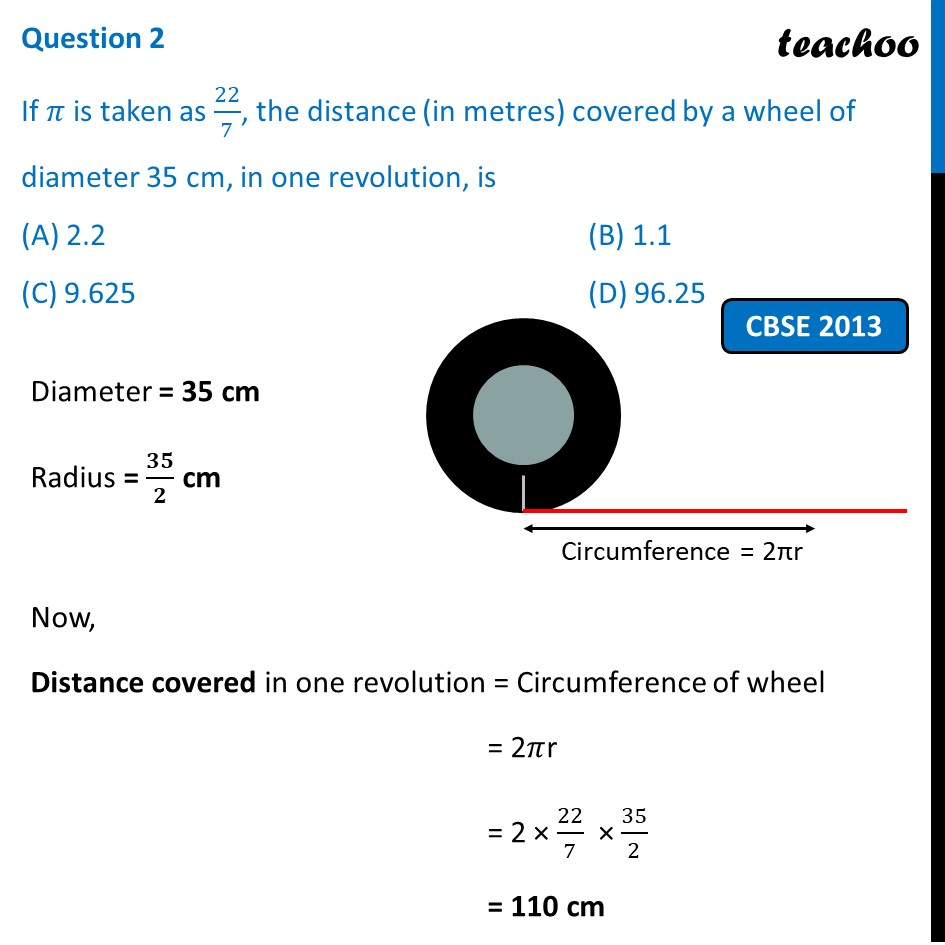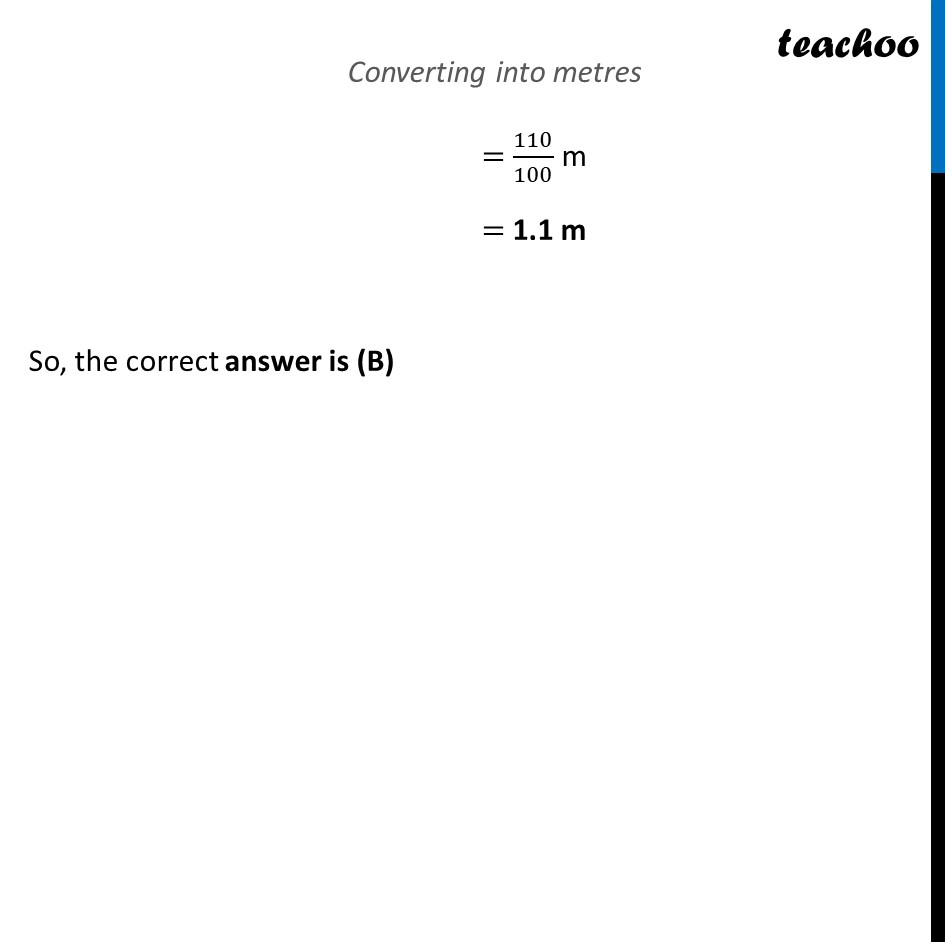## (D) 96.25

This question is similar to Question 15 - CBSE Class 10 Sample Paper for 2022 Boards - Maths Standard [MCQ] - Solutions of Sample Papers for Class 10 Boards1. Chapter 12 Class 10 Areas related to Circles (Term 1)
2. Serial order wise
3. Past Year MCQ

Transcript

Question 2 If 𝜋 is taken as 22/7, the distance (in metres) covered by a wheel of diameter 35 cm, in one revolution, is (A) 2.2 (B) 1.1 (C) 9.625 (D) 96.25 Diameter = 35 cm Radius = 𝟑𝟓/𝟐 cm Now, Distance covered in one revolution = Circumference of wheel = 2𝜋r = 2 × 22/7 × 35/2 = 110 cm Converting into metres =110/100 m = 1.1 m So, the correct answer is (B)